# Using papeR – A short tutorial

This is a short tutorial that covers some of the main features of the R package papeR.

The main goal of the package is to ease statistical reporting and thus to ease reproducible research. By relying on powerful tools such as the Sweave, or the packages knitr and xtable, the package can be easily integrated in existing workflows.

• First of all, the package provides an infrastructure to handle variable labels which are used in all other functions (labels()).

• The package allows to create (complex) summary tables of the data sets (summarize()) and to easily plot the data (plot() for labeled data.frames).

• Finally, the package allows to enhance summary tables of statistical models by (possibly) adding confidence intervals, significance stars, odds ratios, etc. and by separating variable names and factor levels (prettify()).

## Getting started

Before we start, we need to install the package. The package can be easily obtained from CRAN, e.g. via the command

install.packages("papeR")

To install the latest development version, one can use devtools to install packages from GitHub. Therefore we need to install and load devtools before we can install papeR:

install.packages("devtools")
library("devtools")
install_github("hofnerb/papeR")

Now we can load the package

library("papeR")

## The package

### Labeled data frames

To be able to use all features of the package, we first need to create a labeled data frame. We need labeled data frames to use the special plot() function (see below). All other functions do not strictly require labeled data frames but can exploit the labels.

Labels in papeR are stored as attributes of the variables, i.e., each variable in a labeld data frame has an attribute "variable.label", and the data set gets an additional class 'ldf'. Other packages store variable labels differently. E.g. the function read.spss() from the package foreign stores variable labels as a single attribute of the data set. The package papeR is also capable of using these labels. For details see the section “Conversion to labeled data frames”.

#### Setting and extracting labels

If we create a new data.frame we can extract and set variable labels using the function labels(). We use the Orthodont data package nlme throughout this tutorial. First load the data

data(Orthodont, package = "nlme")
## keep the original data set for later use
Orthodont_orig <- Orthodont

To check if the data set is a labeled data set (i.e., of class 'ldf'), we can use

is.ldf(Orthodont)
##  FALSE

Despite the fact that we do not have a labeled data frame, we can query the labels. In this case, we simply get the variable names as no labels were set so far

labels(Orthodont)
##   distance        age    Subject        Sex
## "distance"      "age"  "Subject"      "Sex"

This is a convenient feature, as we thus can relly on the fact that we will always have some variable labels.

To explicitly set labels, which are usually more descriptive than the variable names, we can simply assign a vector of labels. We use some of the information which is given on the help page of the Orthodont data and use it as labels:

labels(Orthodont) <- c("fissure distance (mm)", "age (years)", "Subject", "Sex")

If we now query if Orthodont is a labeled data frame and extract the labels, we get

is.ldf(Orthodont)
##  TRUE
class(Orthodont)
##  "ldf"            "nfnGroupedData" "nfGroupedData"  "groupedData"
##  "data.frame"

We see that by setting variable labels, we also add the class 'ldf' to the data frame. Now, the labels are

labels(Orthodont)
##                distance                     age                 Subject
## "fissure distance (mm)"           "age (years)"               "Subject"
##                     Sex
##                   "Sex"

We can also set or ectract labels for a subset of the variables using the option which, which can either be a vector of variable names or indices. Let’s capitalize the labels of distance and age to make it consitent with Subject and Sex:

## set labels for distance and age
labels(Orthodont, which = c("distance", "age")) <- c("Fissure distance (mm)", "Age (years)")
## extract labels for age only
labels(Orthodont, which = "age")
##           age
## "Age (years)"
## or for the first two variables (i.e., distance and age)
labels(Orthodont, which = 1:2)
##                distance                     age
## "Fissure distance (mm)"           "Age (years)"

#### Conversion to labeled data frames

Instead of manually setting labels, we can simply convert a data frame to a labeled data frame, either with the function as.ldf() or with convert.labels(). Actually, both calls reference the same function (for an object of class data.frame).

While as.ldf() can be seen as the classical counterpart of is.ldf(), the function name convert.labels() is inspired by the fact that these functions either convert the variable names to labels or convert other variable labels to papeR-type variable labels. Hence, these functions can, for example, be used to convert labels from data sets which are imported via the function read.spss() to papeR-type variable labels.

If no variable labels are specified, the original variable names are used.

Orthodont2 <- convert.labels(Orthodont_orig)
class(Orthodont2)
##  "ldf"            "nfnGroupedData" "nfGroupedData"  "groupedData"
##  "data.frame"
labels(Orthodont2)
##   distance        age    Subject        Sex
## "distance"      "age"  "Subject"      "Sex"

### Plotting labeled data frames

For data frames of class 'ldf', there exist special plotting functions:

par(mfrow = c(2, 2))
plot(Orthodont)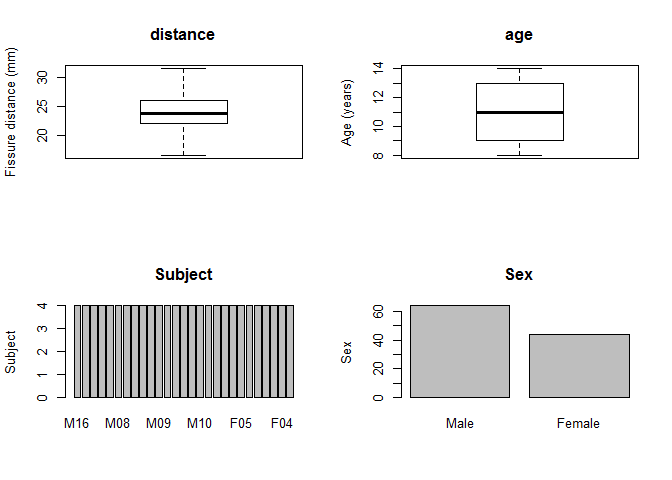As one can see, the plot type is automatically determined based on the data type and the axis label is defined by the labels().

To obtain group comparisons, we can use grouped plots. To plot all variable in the groups of Sex one can use

par(mfrow = c(1, 3))
plot(Orthodont, by = "Sex")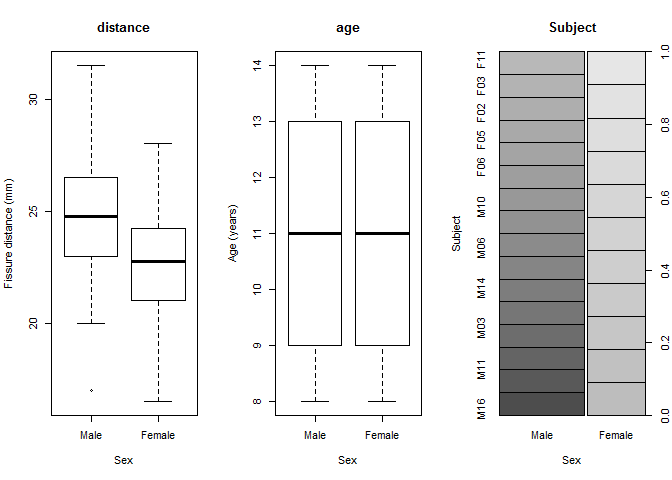We can as well plot everything against the metrical variable distance

par(mfrow = c(1, 3))
plot(Orthodont, with = "distance")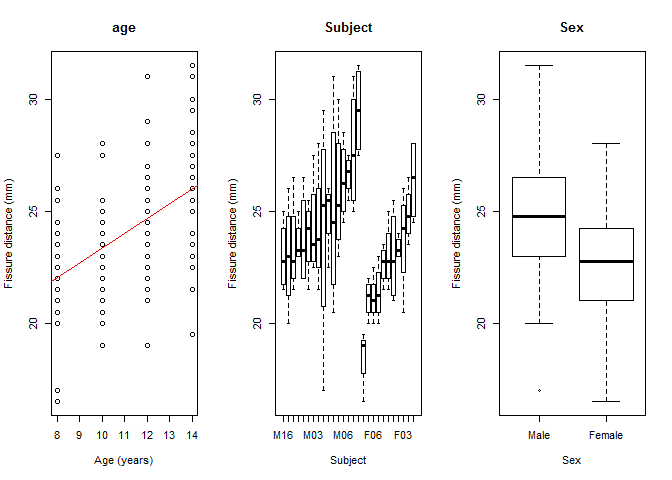To plot only a subset of the data, say all but Subject, against distance and suppress the regression line we can use

par(mfrow = c(1, 2))
plot(Orthodont, variables = -3, with = "distance", regression.line = FALSE)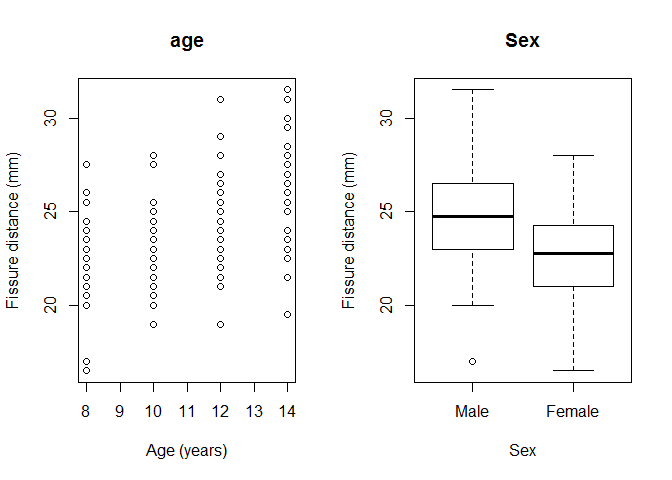Note that again we can use either variable names or indices to specify the variables which are to be plotted.

### Summary tables

One can use the command summarize() to automatically produce summary tables for either numerical variables (i.e., variables where is.numeric() is TRUE) or categorical variables (where is.factor() is TRUE). We now extract a summary table for numerical variables of the Orthodont data set:

data(Orthodont, package = "nlme")
summarize(Orthodont, type = "numeric")
##              N    Mean   SD    Min Q1 Median Q3  Max
## 1 distance 108   24.02 2.93   16.5 22  23.75 26 31.5
## 2      age 108   11.00 2.25    8.0  9  11.00 13 14.0

Similarly, we can extract summaries for all factor variables. As one of the factors is the Subject which has 27 levels, each with 4 observations, we exclude this from the summary table and only have a look at Sex

summarize(Orthodont, type = "factor", variables = "Sex")
##        Level    N    %
## 1 Sex   Male   64 59.3
## 2     Female   44 40.7

Again, as for the plots, one can specify groups to obtain grouped statistics:

summarize(Orthodont, type = "numeric", group = "Sex", test = FALSE)
##               Sex    N    Mean   SD    Min Q1 Median    Q3  Max
## 1 distance   Male   64   24.97 2.90   17.0 23  24.75 26.50 31.5
## 2          Female   44   22.65 2.40   16.5 21  22.75 24.25 28.0
## 3      age   Male   64   11.00 2.25    8.0  9  11.00 13.00 14.0
## 4          Female   44   11.00 2.26    8.0  9  11.00 13.00 14.0

Per default, one also gets tests for group differences:

summarize(Orthodont, type = "numeric", group = "Sex")
##               Sex    N    Mean   SD    Min Q1 Median    Q3  Max   p.value
## 1 distance   Male   64   24.97 2.90   17.0 23  24.75 26.50 31.5    <0.001
## 2          Female   44   22.65 2.40   16.5 21  22.75 24.25 28.0
## 3      age   Male   64   11.00 2.25    8.0  9  11.00 13.00 14.0     1.000
## 4          Female   44   11.00 2.26    8.0  9  11.00 13.00 14.0

### Converting summaries to PDF

So far, we only got standard R output. Yet, any of these summary tables can be easily converted to LaTeX code using the package xtable. In papeR two special functions xtable.summary() and print.xtable.summary() are defined for easy and pretty conversion. In Sweave we can use

<<echo = FALSE, results = tex>>=
xtable(summarize(Orthodont, type = "numeric"))
xtable(summarize(Orthodont, type = "factor", variables = "Sex"))
xtable(summarize(Orthodont, type = "numeric", group = "Sex"))
@

and in knitr we can use

<<echo = FALSE, results = 'asis'>>=
xtable(summarize(Orthodont, type = "numeric"))
xtable(summarize(Orthodont, type = "factor", variables = "Sex"))
xtable(summarize(Orthodont, type = "numeric", group = "Sex"))
@

to get the following PDF output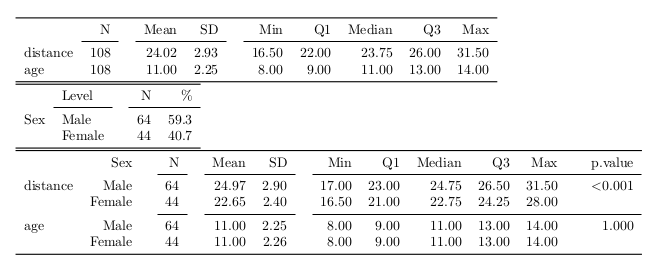LaTeX Output

Note that per default, booktabs is set to TRUE in print.xtable.summary, and thus \usepackage{booktabs} is needed in the header of the LaTeX report. For details on LaTeX summary tables see the dedicated vignette, which can be obtained, e.g., via vignette("papeR\_with\_latex", package = "papeR"). See also there for more details on summary tables in general.

### Converting summaries to Markdown

To obtain markdown output we can use, for example, the function kable() from package knitr on the summary objects:

{r, echo = FALSE, results = 'asis'}
library("knitr")
kable(summarize(Orthodont, type = "numeric"))
kable(summarize(Orthodont, type = "factor", variables = "Sex", cumulative = TRUE))
kable(summarize(Orthodont, type = "numeric", group = "Sex", test = FALSE))


which gives the following results

N Mean SD Min Q1 Median Q3 Max
distance 108 24.02 2.93 16.5 22 23.75 26 31.5
age 108 11.00 2.25 8.0 9 11.00 13 14.0
Level N % $$\sum$$ %
Sex Male 64 59.3 59.3
Female 44 40.7 100.0
Sex N Mean SD Min Q1 Median Q3 Max p.value
1 distance Male 64 24.97 2.90 17.0 23 24.75 26.50 31.5 <0.001
1.1 Female 44 22.65 2.40 16.5 21 22.75 24.25 28.0
2 age Male 64 11.00 2.25 8.0 9 11.00 13.00 14.0 1.000
2.1 Female 44 11.00 2.26 8.0 9 11.00 13.00 14.0

### Prettify model output

To prettify the output of a linear model, one can use the function prettify(). This function adds confidence intervals, properly prints p-values, adds significance stars to the output (if desired) and additionally adds pretty formatting for factors.

linmod <- lm(distance ~ age + Sex, data = Orthodont)
## Extract pretty summary
(pretty_lm <- prettify(summary(linmod)))
##                 Estimate CI (lower) CI (upper) Std. Error   t value
## 1 (Intercept) 17.7067130 15.5014071 19.9120189 1.11220946 15.920304
## 2         age  0.6601852  0.4663472  0.8540231 0.09775895  6.753194
## 3 Sex: Female -2.3210227 -3.2031499 -1.4388955 0.44488623 -5.217115
##   Pr(>|t|)
## 1   <0.001 ***
## 2   <0.001 ***
## 3   <0.001 ***

The resulting table can now be formatted for printing using packages like xtable for LaTeX which can be used in .Rnw files with the option results='asis' (in knitr) or results = tex (in Sweave)

xtable(pretty_lm)

In markdown files (.Rmd) one can instead use the function kable() with the chunk option results='asis'. The result looks as follows:

kable(pretty_lm)
Estimate CI (lower) CI (upper) Std. Error t value Pr(>|t|)
(Intercept) 17.7067130 15.5014071 19.9120189 1.1122095 15.920304 <0.001 ***
age 0.6601852 0.4663472 0.8540231 0.0977589 6.753194 <0.001 ***
Sex: Female -2.3210227 -3.2031499 -1.4388955 0.4448862 -5.217115 <0.001 ***

#### Supported objects

The function prettify is currently implemented for objects of the following classes:

• lm (linear models)
• glm (generalized linear models)
• coxph (Cox proportional hazards models)
• lme (linear mixed models; implemented in package nlme)
• mer (linear mixed models; implemented in package lme4, version < 1.0)
• merMod (linear mixed models; implemented in package lme4, version >= 1.0)
• anova (anova objects)

## Summary and Outlook

The package is intended to ease reporting of standard data analysis tasks such as descriptive statistics, simple test results, plots and to prettify the output of various statistical models.

papeR is under active development. Feature requests, bug reports, or patches, which either add new features or fix bugs, are always welcome. Please use the GitHub page.